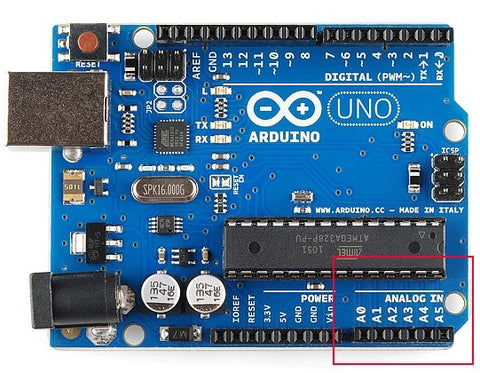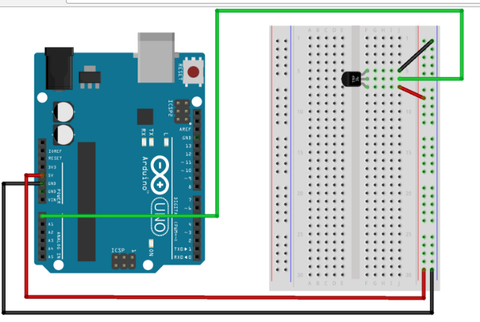How to setup Arduino with room temperature monitoring using LM36 senso – Zero Ohm Electronics

How to setup Arduino with room temperature monitoring using LM36 sensor

Posted by Momen Odeh on

Welcome to Zeroohm Arduino Training series. In this series of Arduino Training, Zeroohm will focus its efforts on helping the community learn more about Arduino fundamentals and basics starting with basic control of LED's, motors, sensors and utilizing of wirless communication. We hope that you find this useful, Please, leave a comment for any questions or comments or reviews!

We provide all our trainings for groups from schools, universities, public sector and private sector in Abu Dhabi, Dubai, Sharjah, Fujirah, RAK or anywhere in UAE. Please,click here to contact us directly for any training inquires.

We are also an authorized Arduino distributor for the UAE, Oman, Qatar, Saudi, Kuwait and Bahrain. We can provide you with any product of your choice. Please, contact us for inquires.

Our Arduino training series:

5. Introduction to IoT using Arduino

Welcome to Zeroohm tutorials !

In this tutorial, you will learn how to integrate the temperature sensor LM36 with Arduino board (any type), and use the Arduino IDE’s serial monitor to display the temperature values.
The TMP36 sensor has a nominal 750 mV at 25°C (about room temperature). This particular sensor has three pins – a positive, a ground, and a signal. This is a linear temperature sensor. A change in temperature of one degree centigrade is equal to a change of 10 millivolts at the sensor output. LM36 can be used for all kinds of automation tasks where you would like to know or control the ambient temperature.
Required Parts:
•    1x Arduino board (UNO or any type)
•    1X Temperature sensor LM36
•    5X jumpers wiresFig2:   Arduino UNOFig3: LM36 temperature sensorFig 4: Jumpers Wires

Technical details of electronic Parts:
LM36 sensor- see fig 3:
•    Low voltage operation (2.7 V to 5.5 V)
•    Calibrated directly in °C
•    10 mV/°C scale factor (20 mV/°C on TMP37)
•    ±2°C accuracy over temperature (typ)
•    ±0.5°C linearity (typ)
•    Stable with large capacitive loads
•    Specified −40°C to +125°C, operation to +150°C
•    Less than 50 μA quiescent current
•    Shutdown current 0.5 μA max
•    Low self-heating
•    Qualified for automotive applicationsFig 5: the graph of temperature versus output voltage

Arduino UNO - see fig 2:
The ARDUINO UNO is the most robust board that you can start with it to implement very useful projects and circuits.
Arduino Uno is a microcontroller board based on the ATmega328P. Arduino UNO has 14 digital input/output pins (of which six can be use as PWM outputs), six analog inputs, a 16 MHz quartz crystal, a USB connection, a power jack, an ICSP header and a reset button. It contains everything needed to support the microcontroller; simply connect it to a computer with a USB cable or power it with an AC-to-DC adapter (5V output) or battery 9V to get started. The recommended input voltage is 7-12 volt. Arduino can be use in a wide range of application and control systems design. The following table shows the technical specification of Arduino UNO.
Microcontroller      Atmega328p
Operating Voltage        5V

Input Voltage (recommended)    7-12V
Digital I/O Pins        14 (of which 6 provide PWM output

PWM Digital I/O Pins    6
DC Current per I/O Pin        20 mA

DC Current for 3.3V Pin        50 mA

Flash Memory    32 KB (ATmega328P)
of which 0.5 KB used by boot loader
SRAM    2 KB (ATmega328P)
EEPROM    EEPROM
Clock Speed    16 MHz
LED_BUILTIN    13
Length        68.6 mm

Weight        25 g

Important Note:

Make sure to connect the LM36 sensor in the correct polarize as showing below.Fig 5:  LM36 sensor Terminals
The LM36 is an analog sensor, which means that the output for it is an analog voltage, in the following paragraphs analog functions will be explained.
An Analog to Digital Converter (ADC) is a very useful feature that converts an analog voltage on a pin to a digital number. By converting from the analog signals to the digital signals, we can begin to use electronics to interface to the analog world around us.
The ADC on the Arduino is a 10-bit ADC meaning it has the ability to detect 1,024 (210) discrete analog levels. Some microcontrollers have 8-bit ADCs (28 = 256 discrete levels) and some have 16-bit ADCs (216 = 65,535 discrete levels) depending on microcontroller family. See the figure below:
Not every pin on a microcontroller has the ability to do analog to digital conversions. On the Arduino board, these pins have an ‘A’ in front of their label (A0 through A5) to indicate these pins can read analog voltages.The analog read () command converts the input voltage range, 0 to 5 volts, to a digital value between 0 and 1023. A circuit inside the microcontroller called an analog-to-digital converter or ADC does this.
The TMP36 and does not act like a resistor. Because of that, there is really only one way to read the temperature value from the sensor, and that is plugging the output pin directly into an Analog (ADC) input (note that any analog input pin can be use).
The Arduino UNO contains six channels of analog input, 10-bit analog to digital converter. This means that it will map input voltages between 0 and 5 volts into integer values between 0 and 1023. This yields a resolution between readings of 5 volts / 1024 units or, .0049 volts (4.9 mV) per unit. The input range and resolution can be changed using analog reference ()  . It takes about 100 microseconds (0.0001 s) to read an analog input, so the maximum reading rate is about 10,000 times a second. Note the following points for analog reference ()  .
If you are using a 5V Arduino (UNO, mega.), you will want to use this formula:
this formula converts the number 0-1023 from the ADC into 0-5000mV (= 5V)
If you are using a 3.3V Arduino (for example Arduino Pro Mini 3.3V), you will want to use this formula:
this formula converts the number 0-1023 from the ADC into 0-3300mV (= 3.3V)
Then, to convert millivolts into temperature, use this formula:
Temperature = (voltage - 0.5) * 100;        as showing the programing code.

Experiment Steps:
The circuit:
The following block diagram showing the hardware circuit connection between LM36 and Arduino UNO.    (Note that any Arduino board can be use in this tutorial).Fig7: block diagram of LM36 sensor and Arduino UNO
When you connect, the circuit make sure to connect the correct voltage terminal to LM36 sensor. Arduino board has voltage source 5V and GND (ground) it can be used to feed the sensor with required power. The signal terminal for LM36 can be connected to any analog input ports in Arduino board.

Coding:
Open Arduino IDE software and write the following code, before using the serial monitor, you must call Serial. Begin () to initialize it. 9600 is the “baud rate”, or communications speed. When two devices are communicating with each other, both must be set to the same speed.
Serial. Print () will print everything on the same line. Serial.println () will move to the next line. By using both of these commands together, you can create easy-to-read printouts of text and data.
The following code should beD verified using Arduino IDE itself before uploading to the Arduino to detect any syntax errors.

void setup() {                      //  the main setup function function  in the program
Serial.begin(9600); }         //  initialize  the speed of serial communication between pc and Arduino .
void loop() {                                          //  the program body  function
float voltage, degreesC, degreesF;  //  define the variables
voltage = getVoltage(tempPin);
degreesC = (voltage - 0.5) * 100.0;     //  this equation form lm36 sensor data sheet
degreesF = degreesC * (9.0/5.0) + 32.0;
Serial.print("voltage: ");    //  print the values of output voltage
Serial.print(voltage);
Serial.print(" deg C: ");     // print the values of temperature in centigrade (C)
Serial.print(degreesC);
Serial.print(" deg F: ");   //  print the values of temperature in Fahrenheit (F)
Serial.println(degreesF);
delay(1000); }
float getVoltage(int pin) {

Result:
The following result it will show in the Arduino IDE serial monitor after uploading the above code, note that  sensor values is depending on the room temperature .
For example:
Voltage: 0.73 deg C: 23.24 deg F: 73.84
Voltage: 0.73 deg C: 23.24 deg F: 73.84
Voltage: 0.73 deg C: 22.75 deg F: 72.96
Voltage: 0.73 deg C: 23.24 deg F: 73.84
Voltage: 0.73 deg C: 23.24 deg F: 73.84

You can get any of these items from our online store.

We will ship them to your door steps in UAE, Qatar or Oman.